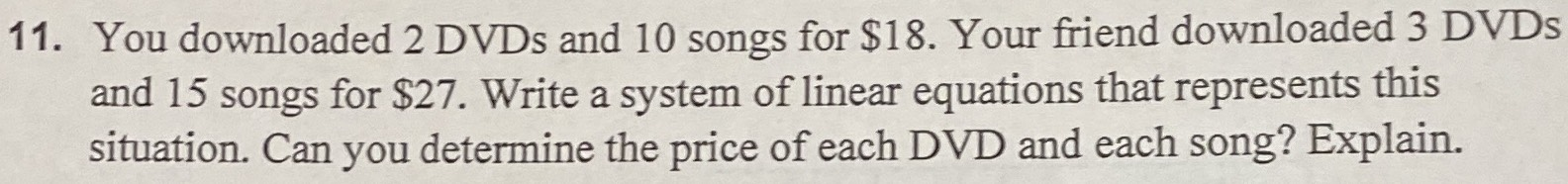### ¿Todavía tienes preguntas de matemáticas?

Pregunte a nuestros tutores expertos
Algebra
Pregunta11. You downloaded $$2$$ DVDs and $$10$$ songs for $$\ 18$$ . Your friend downloaded $$3$$ DVDs and $$15$$ songs for $$\ 27$$ . Write a system of linear equations that represents this situation. Can you determine the price of each DVD and each song? Explain.

$$2x+ 10y = 18\\ 3x+ 15y = 27$$